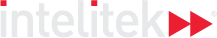### Course Overview

This course explores analytical geometry, coordinate systems and equations of lines. The slope intercept equations, which are already familiar from previous studies, are reintroduced, along with parallel and intersecting lines. The course concludes with a discussion on variation, as well as hypothesis testing and the concept of t-tests.

HOURS OF INSTRUCTION: 15

### Course Outline

• Introduction to Analytical Geometry
• Equation of a Straight Line
• Intersection of Lines
• Variation
• Introduction to Hypotheses Testing and the T-test